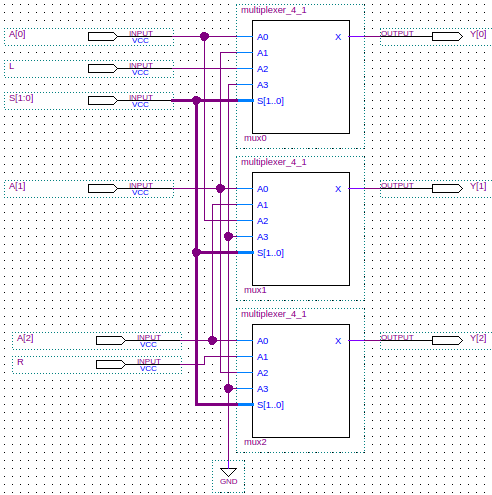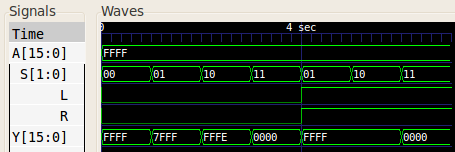# Shifter

A shifter is a combinational logic circuit which shifts the bits of a value one place to the left or right. The shifter's function is similar to that of a bidirectional shift register. However, the register is a sequential logic circuit and this makes it unsuitable to be used in a function unit.

The basic n-bit shifter design comprises n 2-to-4 multiplexers, one multiplexer per input bit. If we have an n-bit input `A`, then the multiplexer for bit m is configured as follows:

Input line Input Value
`0` `A[m]`
`1` `A[m+1]`
`2` `A[m-1]`
`3` `0` (Unused)

The table below shows the meaning of the selection binary code input to the multiplexer in such a configuration:

Selection code Meaning
`00` No shift
`01` Shift right
`10` Shift left
`11` Zero output

The only problem with this is what happens at the most and least significant bits. Upon a left shift, we need to give an input to tell the shifter what the new least significant bit should be as there is no `A[-1]`. Let's call this `L`. Similarly, Upon a right shift, we need to give an input to tell the shifter what the new most significant bit should be as there is no `A[n+1]`. Let's call this `R`. This is probably easier to understand if we rewrite the multiplexer configuration table for the least significant bit case (bit `0`):

Input line Input Value
`0` `A`
`1` `A`
`2` `L`
`3` `0` (Unused)

And the following table is for the most significant bit (bit `n`):

Input line Input Value
`0` `A[n]`
`1` `R`
`2` `A[n-1]`
`3` `0` (Unused)

## Example

The schematic for a shifter is shown below. To save space, a 3-bit shifter design is shown. Here the shifted output is the 3-bit value `Y`. The 2-bit input value `S` is the multiplexer selector.## Verilog

Below is the Verilog code for a structural model of a 16-bit shifter. The code is also shown for the multiplexer.

```	```
module shifter_16(Y, A, S, L, R);
output [15:0] Y;  // The shifted result.
input [15:0]  A;  // The value to be shifted.
input [1:0] 	 S;  // The direction of the shift.
input 	 L;  // The leftmost bit to shift in.
input 	 R;  // The rightmost bit to shift in.

multiplexer_4_1 #(1) mux0(Y, A, A, L, 1'b0, S, S);
multiplexer_4_1 #(1) mux1(Y, A, A, A, 1'b0, S, S);
multiplexer_4_1 #(1) mux2(Y, A, A, A, 1'b0, S, S);
multiplexer_4_1 #(1) mux3(Y, A, A, A, 1'b0, S, S);
multiplexer_4_1 #(1) mux4(Y, A, A, A, 1'b0, S, S);
multiplexer_4_1 #(1) mux5(Y, A, A, A, 1'b0, S, S);
multiplexer_4_1 #(1) mux6(Y, A, A, A, 1'b0, S, S);
multiplexer_4_1 #(1) mux7(Y, A, A, A, 1'b0, S, S);
multiplexer_4_1 #(1) mux8(Y, A, A, A, 1'b0, S, S);
multiplexer_4_1 #(1) mux9(Y, A, A, A, 1'b0, S, S);
multiplexer_4_1 #(1) mux10(Y, A, A, A, 1'b0, S, S);
multiplexer_4_1 #(1) mux11(Y, A, A, A, 1'b0, S, S);
multiplexer_4_1 #(1) mux12(Y, A, A, A, 1'b0, S, S);
multiplexer_4_1 #(1) mux13(Y, A, A, A, 1'b0, S, S);
multiplexer_4_1 #(1) mux14(Y, A, A, A, 1'b0, S, S);
multiplexer_4_1 #(1) mux15(Y, A, R, A, 1'b0, S, S);
endmodule // shifter_16

module multiplexer_4_1(X, A0, A1, A2, A3, S1, S0);
parameter WIDTH=16;     // How many bits wide are the lines

output [WIDTH-1:0] X;   // The output line

input [WIDTH-1:0]  A3;  // Input line with id 2'b11
input [WIDTH-1:0]  A2;  // Input line with id 2'b10
input [WIDTH-1:0]  A1;  // Input line with id 2'b01
input [WIDTH-1:0]  A0;  // Input line with id 2'b00
input 	      S0;  // Least significant selection bit
input 	      S1;  // Most significant selection bit

assign X = (S1 == 0
? (S0 == 0
? A0       // {S1,S0} = 2'b00
: A1)      // {S1,S0} = 2'b01
: (S0 == 0
? A2       // {S1,S0} = 2'b10
: A3));    // {S1,S0} = 2'b11
endmodule // multiplexer_4_1
```
```

When given test inputs, the 16-bit shifter generated the following waveform:## References

Mano, M. Morris, and Kime, Charles R. Logic and Computer Design Fundamentals. 2nd Edition. Prentice Hall, 2000.